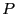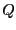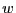Next: Safe evaluation of a Up: Utilities Previous: Centered form   Contents

Unitary polynomial

Letbe a polynomial and be maxroot the maximal modulus of the root of. Fromwe may derive a polynomialsuch that the roots ofhave a modulus lower or equal to 1 and ifis a root ofthen maxrootis a root of. The calculation of the coefficients ofmay be done with the procedure

int Unit_Polynom(int Degree,INTERVAL_VECTOR &Coeff,
double maxroot,INTERVAL_VECTOR &CoeffU)
where Coeff are the coefficients ofand CoeffU the coefficients of. This procedure returns 1 if the calculation has been successfull, 0 otherwise.

Jean-Pierre Merlet 2012-12-20